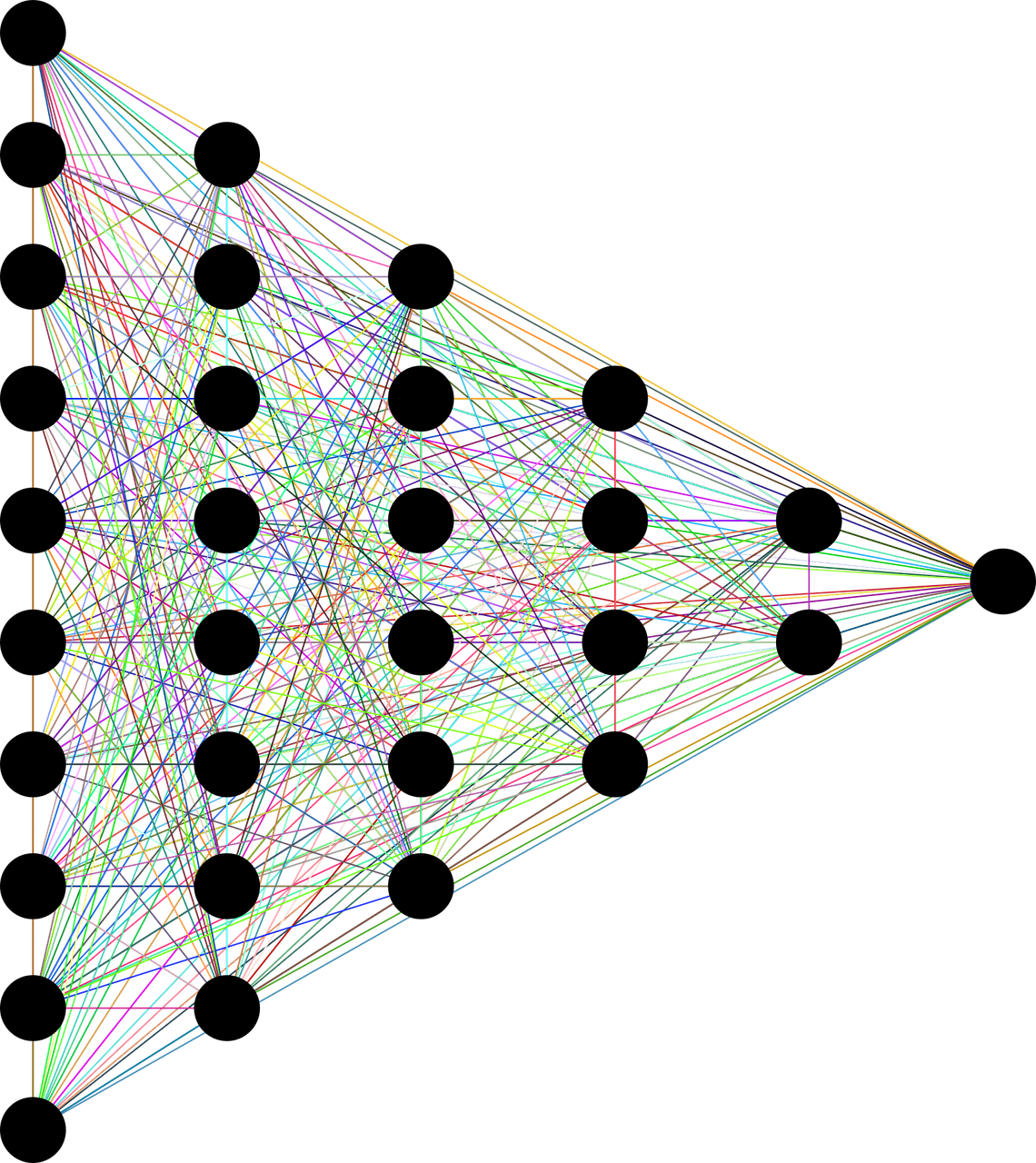# What is Deep Learning, How Deep Learning Works, Algorithms used in Deep Learning

##What is Deep learning?

Deep learning is a subfield of machine learning and artificial intelligence which copy the way the human brain gains certain knowledge. It is a powerful set of techniques for learning in neural networks. The meaning of the ‘Deep’ in deep learning is referring to the depth of the layers in a neural network. A deep learning model is created with the help of a neural network that consists of artificial neurons.

A neural network is divided into three major layers input layer( it is the first layer of the neural network where we get input), hidden layer (it is the second layer of our neural network which help us to us accurate input on how much-hidden layer in our model that much accurate output we get) and output layer (This is our third or last layer where we get output).

## How Deep learning works

Deep learning methods use neural network architectures that’s why the deep learning model is also called a deep neural network. A normal neural network model only consists of three layers Input layer, the hidden layer, and the output layer but a deep network can consist of as many as 150 hidden layers and the main thing is how much-hidden layers in our model that much accurate output we get.

The deep learning model is trained by lots of data and after the training, it’s able to give us an accurate output of deep neural networks consisting of multiple layers of interconnected nodes, each neuron is connected to the previous layer neurons to refine and optimize the prediction or categorization. The input and output layers of a deep network are called visible layers. The input layer is where the deep learning model is get the data for processing, and the output layer is where the final prediction or classification gets.

We gave input from the input layer and then with the help of deep learning algorithms our model is trained ( Algorithms are like Conventional neural networks, Radial-Bias function, Multi-layer Perceptron, etc and the algorithms also eliminate the manual feature extraction). and then our model gives us an accurate output in this way deep learning is work. We also use backpropagation algorithms to calculate errors in predictions. (Backpropagation algorithms are like gradient descent, Boltzmann learning, Hebbian learning, etc)

## Some Algorithms used in Deep Learning

• Convolutional Neural Networks.
• Feed Forward Network.
• Feed Back Network.
• Multi-layer Perceptrons
• Self Organizing Maps.

## Convolutional Neural Network

The convolutional neural network consists of multiple layers which mainly used for image processing and object detection. CNN first, it is developed in 1988 that time it was called LeNet. It is used for recognizing characters like ZIP codes and digits and CNN is also widely used to Identify satellite images, Medical images, forecast time series, etc.

## Feed-forward Network

In a feed-forward network, the signal is traveled in only one direction from the input layer to the hidden layer to the output layer, and single only travels in one direction in this network that’s why is not give us an accurate input as other algorithms give us and they have fixed input and output. It is mostly used in pattern generation, pattern recognition, pattern classification, etc.

## Feed-back Network

In a feedback network, the signal is traveled in both directions so that’s why a feedback network becomes very powerful it gives more accurate output to the comparison of feed-froward network and the single can travel in both directions so due to this it also becomes complicated and the state in feedback network keeps changing until they do not reach an equilibrium point. Feedback neural network architecture is also referred to as an interactive or recurrent network. it is used in content-addressable memories.

The radial basis function is composed of an input layer, hidden layer, and output layer in Radial basis is strictly limited to only having one hidden layer.

On a Radial basis, the input layer acts as a source node that connects the network to its environment.

The Hidden layer provides a set of units of basis function and high dimensionality.

The output layer provides a linear combination of hidden functions that perform a simple weighted sum with a linear output.

In Radial Basis function networks are used for function approximation (matching a real number) then this output is used is fine however if pattern classification is required then a hard-limiter or sigmoid function could be placed on the output of neurons to get 0/1 output values.

## Multi-layer perceptron

A multi-layer perceptron is a feed-forward artificial neural network that generates a set of outputs from a set of inputs and an MLP is characterized by several layers of input nodes connected as a directed graph between the input and output.

MLP has also used backpropagation for training the network and MLP is a deep learning method as we all know in this each node apart from the input node has a non-linear activation function which helps to reduce calculation errors and MLP is widely used for solving problems which require supervised learning and are linearly nonseparable problems like XOR gate. Some application that used MLP is Speech recognition, Image recognition Machine translation, etc.

## Self Organizing Maps

• The self-organizing technique is developed by T.kohonen in 1982. SOM is called ‘Self-organizing’ because there is no supervision required during the learning it learns on its own with the help of unsupervised learning. SOM is trained our network through competitive learning algorithms to map multidimensional data into lower-dimensional which allows easy interception for complex problems.
• Multidimensional data visualization technique.
• Reduces the dimensions of data.
• Trained by unsupervised learning.
• Group similar data items together with the help of clustering.
• Mapping multidimensional data onto a 2D map while preserving proximity relationships as well as possible.